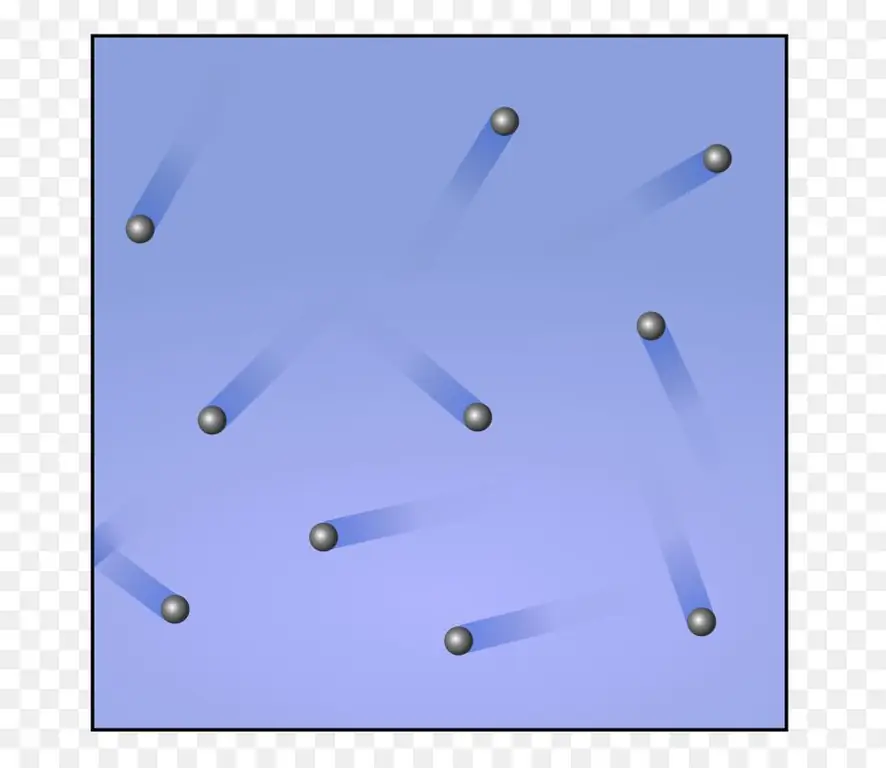Science 2023The equation of state for an ideal gas. Isoprocesses in gases

The gaseous state of the matter around us is one of the three common forms of matter. In physics, this fluid state of aggregation is usually considered in the approximation of an ideal gas. Using this approximation, we describe in the article possible isoprocesses in gases.

## Ideal gas and the universal equation to describe it

An ideal gas is one whose particles do not have dimensions and do not interact with each other. Obviously, there is not a single gas that exactly satisfies these conditions, since even the smallest atom - hydrogen, has a certain size. Moreover, even between neutral noble gas atoms, there is a weak van der Waals interaction. Then the question arises: in what cases can the size of gas particles and the interaction between them be neglected? The answer to this question will be the observance of the following physico-chemical conditions:

• low pressure (about 1 atmosphere and below);
• high temperatures (around room temperature and above);
• chemical inertness of molecules and atomsgas.

If at least one of the conditions is not met, then the gas should be considered real and described by a special van der Waals equation.

The Mendeleev-Clapeyron equation must be considered before studying isoprocesses. The ideal gas equation is its second name. It has the following notation:

PV=nRT

That is, it links three thermodynamic parameters: pressure P, temperature T and volume V, as well as the amount n of the substance. The symbol R here denotes the gas constant, it is equal to 8.314 J / (Kmol).

## What are isoprocesses in gases?

These processes are understood as transitions between two different states of the gas (initial and final), as a result of which some quantities are preserved and others change. There are three types of isoprocess in gases:

• isothermal;
• isobaric;
• isochoric.

It is important to note that all of them were experimentally studied and described in the period from the second half of the 17th century to the 30s of the 19th century. Based on these experimental results, Émile Clapeyron in 1834 derived an equation that is universal for gases. This article is built the other way around - applying the equation of state, we obtain formulas for isoprocesses in ideal gases.

## Transition at constant temperature

It's called an isothermal process. From the equation of state of an ideal gas, it follows that at a constant absolute temperature in a closed system, the product must remain constantvolume to pressure, i.e.:

PV=const

This relationship was indeed observed by Robert Boyle and Edm Mariotte in the second half of the 17th century, so the equality currently recorded bears their names.

Functional dependencies P(V) or V(P), expressed graphically, look like hyperbolas. The higher the temperature at which the isothermal experiment is carried out, the greater the product PV.

In an isothermal process, a gas expands or contracts, doing work without changing its internal energy.

## Transition at constant pressure

Now let's study the isobaric process, during which the pressure is kept constant. An example of such a transition is the heating of the gas under the piston. As a result of heating, the kinetic energy of the particles increases, they begin to hit the piston more often and with greater force, as a result of which the gas expands. In the process of expansion, the gas performs some work, the efficiency of which is 40% (for a monatomic gas).

For this isoprocess, the equation of state for an ideal gas says that the following relation must hold:

V/T=const

It is easy to obtain if the constant pressure is transferred to the right side of the Clapeyron equation, and the temperature - to the left. This equality is called Charles' Law.

Equality indicates that the functions V(T) and T(V) look like straight lines on the graphs. The slope of the line V(T) relative to the abscissa will be the smaller, the greater the pressureP.